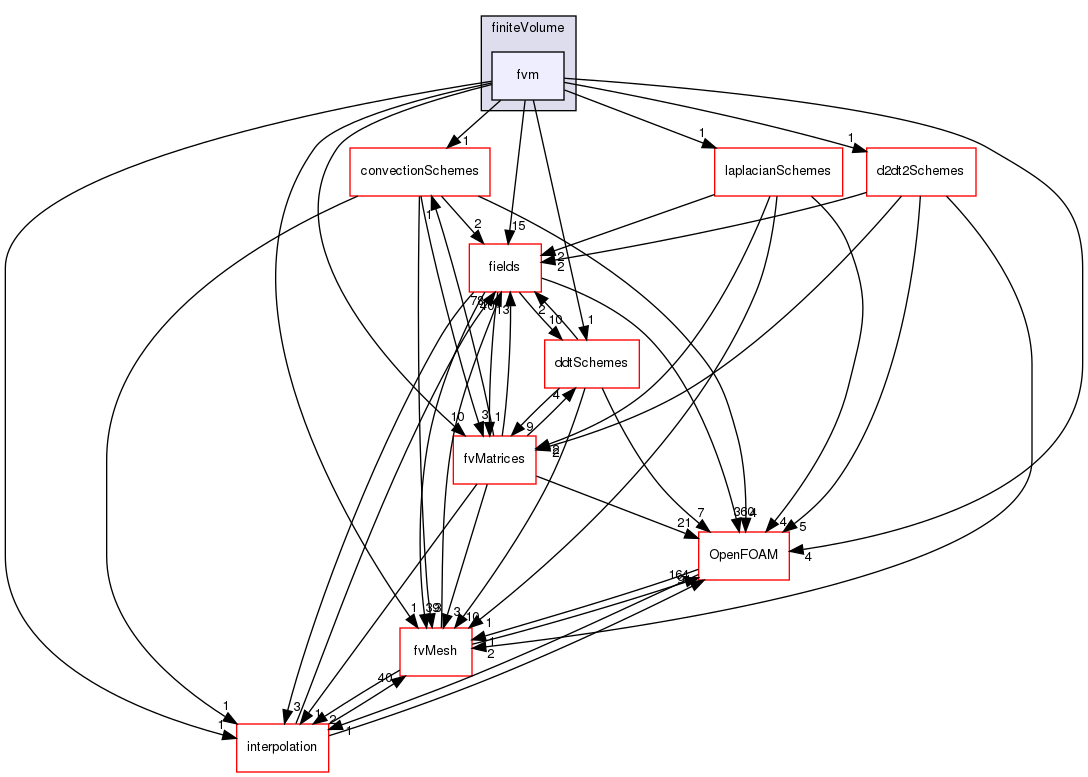The OpenFOAM Foundation
fvm Directory Reference
Directory dependency graph for fvm:## Files

file  fvm.H [code]

file  fvmD2dt2.C [code]

file  fvmD2dt2.H [code]
Calculate the matrix for the second-order temporal derivative.

file  fvmDdt.C [code]

file  fvmDdt.H [code]
Calculate the matrix for the first temporal derivative.

file  fvmDiv.C [code]

file  fvmDiv.H [code]
Calculate the matrix for the divergence of the given field and flux.

file  fvmLaplacian.C [code]

file  fvmLaplacian.H [code]
Calculate the matrix for the laplacian of the field.

file  fvmSup.C [code]

file  fvmSup.H [code]
Calculate the matrix for implicit and explicit sources.